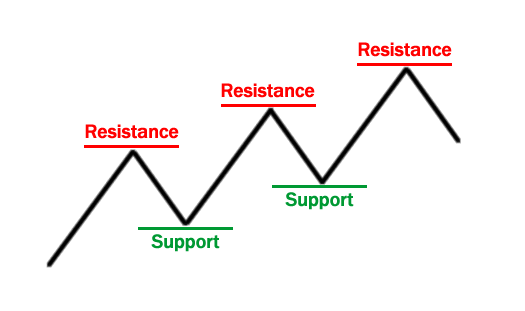Fibonacci studies encompass a series of technical analysis tools based on Fibonacci numbers and ratios representing geometric laws of nature and human behavior, applied to financial markets.

Fibonacci numbers and Fibonacci ratios are used by a wide range of traders to spot potential turning points in the market.

## Fibonacci Series

The Fibonacci series was introduced by an Italian mathematician, Leonardo Pisano Bogollo who was also known as Fibonacci (son of Bonacci), in his 1202 manuscript Liber Abaci.

The series is derived by finding the sum of the two preceding numbers in the series, with 0 and 1 being the seed numbers, or starting point, in the series.

With 0 and 1 being the first two seed numbers, the third number in the series is the sum of 0 and 1 (0 + 1), which is 1.

The fourth number in the series is the sum of the second and third numbers in the series, namely, 1 + 1, and so forth.

The first few numbers in the series thus are:
0, 1, 1, 2, 3, 5, 8, 13, 21, 34, 55, 89, 144, 233, 377, 610, 987, ...

## Fibonacci Ratios

As the numbers in the series increase towards infinity, mathematical relationships, expressed as ratios, appear between the numbers.

For example, the ratio between consecutive numbers tends to oscillate closer and closer towards 1.618034, which is known as the golden ratio or the golden section or the golden mean and is denoted by the upper-case Greek letter Phi Φ in mathematics. This ratio is reached by dividing a number in the summation series by the number that precedes it: 233 ÷ 144 = 1.618055

The inverse of this ratio is 0.618034, which is denoted by the lower-case Greek letter phi φ in mathematics. This ratio is reached by dividing a number in the summation series by the number that succeeds it: 144 ÷ 233 = 0.618025

In general, these ratios are used to predict levels of support and resistance through relationships between Fibonacci numbers. These are the most used Fibonacci ratios:

• 0.618 (61.8%): A Fibonacci number divided by the number that follows is approximately 0.618.

• 0.382 (38.2%): A Fibonacci number divided by the number occupying two later positions is approximately 0.3820.

• 0.2360 (23.60%): A Fibonacci number divided by the number occupying three later positions is approximately 0.2360.

• 0.764 (76.4%): Obtained by adding the difference of 38.2% and 23.6% to 61.8%, that is, 76.4% = 61.8% + (38.2% – 23.6%).

• 0.5 (50%): Half the progress of the main trend. It’s the average between 38.2% and 61.8%.

• 0.0 (0%): Beginning of a market movement.

• 1.9 (100%): End of a market movement.

To understand Fibonacci studies, it’s necessary to remember the concept of support and resistance.• Support: The troughs (or lows). These are the points at which the buying interest is strong enough to overcome the selling pressure. As a result, the decline is suspended and the price rises again.

• Resistance: The peaks (or highs). These represent a price level or area above the market where the selling pressure exceeds the buying pressure.